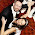## Sunday, 22 April 2012

### cos^2(θ) + sin^2(θ) = 1

I was doing an A-level maths paper when I found this question:
Use this triangle to prove cos2θ + sin2θ = 1. For what values of θ is this prove valid?
My feelings about proof questions are conflicted. When I can see how it works, and I can find an elegant solution, I absolutely love them. Being able to show why something works cements the idea to me that there is a reason for why formulas work for any value you put in, or why you can substitute one formula for another and still come out with the same answer. However, when I can't see how all of the pieces fit together to make a proof, it really frustrates me. I guess that's part of being a mathematician, not knowing how the pieces fit together to start with, but persevering with it until they do.

As it happens, this is a proof question that I could fit together, so I am going to share it here. In my opinion, this is quite an easy one, but easy is subjective, so maybe someone will find it impressive anyway XD

1. Because this triangle is right-angled, I can use trigonometry to show that cosθ = b/c and sinθ = a/c

2. If I square both of them, I end up with cos2θ = b2/c2 and sin2θ = a2/c2

3. Next I add the two together to give cos2θ + sin2θ = (a2 + b2)/c2

4. Pythagoras says: a2 + b2 = cso (a2 + b2)/c2 = 1

5. Therefore cos2θ + sin2θ = 1

For the second part of the question, the proof is only valid for θ < 90o because if θ > 90o, ABC wouldn't be a right-angled triangle.

So that's all there is to the proof. Like I said, I think that one is quite nice. And now to leave you with an interesting fact about the Pythagoras Theorem. In China, it's named after mathematician Shang Gao, who came up with it independently from Pythagoras about 2,200 years ago (300 years after Pythagoras).

#### 1 comment:

1.brain hurts